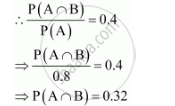Share

# If P(A) = 0.8, P(B) = 0.5 and P(B|A) = 0.4, find P(A ∩ B) - CBSE (Commerce) Class 12 - Mathematics

#### Question

If P(A) = 0.8, P(B) = 0.5 and P(B|A) = 0.4, find P(A ∩ B)

#### Solution

It is given that P(A) = 0.8, P(B) = 0.5, and P(B|A) = 0.4

P (B|A) = 0.4Is there an error in this question or solution?

#### Video TutorialsVIEW ALL 

Solution If P(A) = 0.8, P(B) = 0.5 and P(B|A) = 0.4, find P(A ∩ B) Concept: Conditional Probability.
S